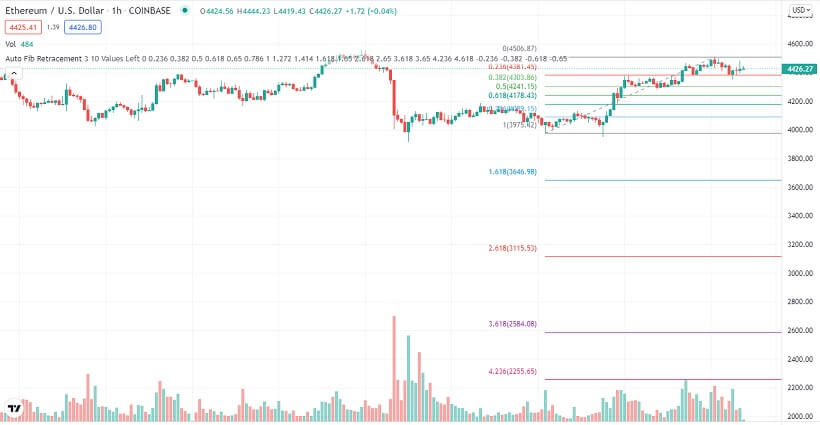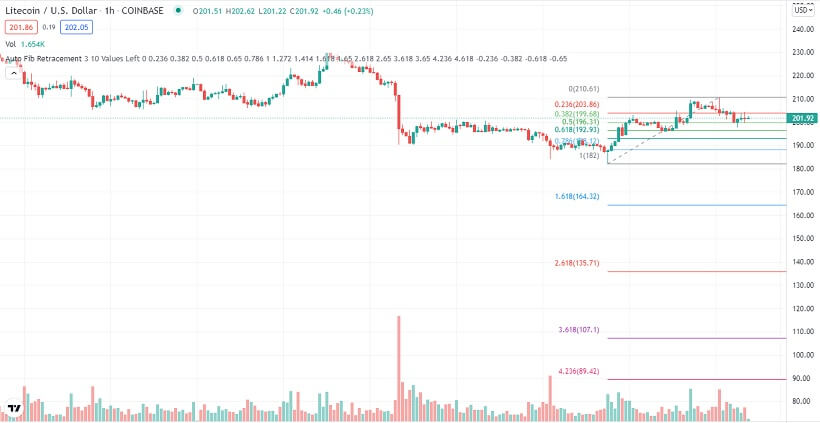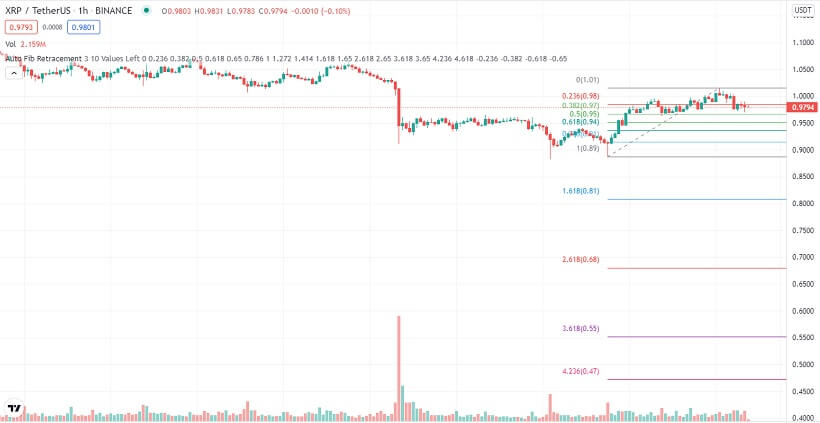# Ethereum, Litecoin, & Ripple XRP Technical Analysis –Nov 30th

## Crypto majors bounce off key support levels after an early morning correction

Last Updated November 30th 2021

## Ethereum

Ethereum was bullish for the better part of Monday. By the end of the day, it was up by 3.2%.

Ethereum started Monday trading bullish, a continuation of the momentum that started over the weekend.

By hour two of the day, Ethereum had pushed through the 50.0% Fibonacci resistance at \$4255.53.

Momentum accelerated and just before daybreak, it had pushed through the 38.2% Fibonacci resistance at \$4326.23.

However, buying volumes dropped at this resistance, and saw Ethereum trade in a range around it until early evening.

An increase in buying momentum across the broader market saw Ethereum rally off the 38.2% Fibonacci all through the evening.

Towards the end of the day, Ethereum pushed through the 23.6% Fibonacci resistance at \$4411.65.

However, there was a minor correction at the 23.6% Fibonacci resistance, in the last two hours of the day.

Ethereum started Tuesday trading with the bullish momentum that had built up on Monday evening.

Just before daybreak, Ethereum came close to hitting the \$4500 minor resistance but failed.

This led to a sharp correction that saw Ethereum breach the 23.6% Fibonacci, then support at \$4441.65, and come close to testing the 38.2% Fibonacci support at \$4326.23.

At the time of going to press, Ethereum was trading slightly above the 23.6% Fibonacci support at \$4326.23.### A glance at the day ahead

In the day, the key levels to watch are the 23.6% Fibonacci support at \$4411.65 and the \$4500 minor resistance.

If bulls take control and push Ethereum through the \$4500 resistance, the next key level to watch would be the multi-week resistance at \$4551.57.

If this resistance is broken, then Ethereum could retest its all-time highs of \$4800 in the day.

On the other hand, if bears take control and push Ethereum through the 23.6% Fibonacci support, the next key level to watch would be the 38.2% Fibonacci support at \$4326.23.

If the 38.2% Fibonacci support is broken, then prices below \$4200 could be seen in the day.

However, if volumes drop in the day, Ethereum could range between the 23.6% Fibonacci support at \$4411.65 and the \$4500 minor resistance.

### A glance at the technicals

Key resistance: \$4500 minor resistance

Key support: 23.6% Fibonacci at \$4411.65

## Litecoin

Litecoin was bullish for the better part of Monday. By the end of the day, it was up by about 2.5%.

Litecoin started Monday trading bullish, a continuation of the momentum it had built up in late Sunday trading.

However, just 3-hours into the day, Litecoin hit the 38.2% Fibonacci resistance at \$200.64.

Without much support from the broader market, Litecoin bulls lost momentum at this resistance.

What followed was a correction that saw Litecoin hit weekly support at \$195.90 by mid-morning.

Litecoin bounced off this support, and by late afternoon, rallied through the 38.2% Fibonacci resistance at \$200.64.

Momentum accelerated and by late evening, Litecoin had pushed through the 50.0% Fibonacci resistance at \$206.27.

However, bullish momentum waned at the 50.0% Fibonacci resistance, and in the last three hours of the day, Litecoin was in a correction.

Litecoin started Tuesday trading in a correction, a continuation of the selloff that started in late Monday trading.

At the time of going to press, Litecoin had pushed through the 38.2% Fibonacci support at \$200.64, and the selloff was accelerating.### A glance at the day ahead

In the day, the key levels to watch are the 38.2% Fibonacci support at \$200.64 and the 50.0% Fibonacci resistance at \$206.27.

If bulls take control and push Litecoin through the 50.0% Fibonacci resistance at \$206.27, the next key level to watch would be the 61.8% Fibonacci resistance at \$212.02.

If this resistance is broken, then Litecoin could test prices above \$218 in the day.

On the other hand, if bears take control and push Litecoin through the 38.2% Fibonacci support at \$200.64, the next key level to watch would be the multi-week support at \$195.90.

If the multi-week support is broken, then prices below \$190 could be seen in the day.

However, if volumes drop in the day, Litecoin could range between the 38.2% Fibonacci support at \$200.64 and the 50.0% Fibonacci resistance at \$206.27.

### A glance at the technicals

Key resistance: 50.0% Fibonacci at \$206.27

Key support:  38.2% Fibonacci at \$200.64

## Ripple XRP

Ripple XRP was bullish for the better part of Monday. By the end of the day, it was up by about 2.5%.

XRP started Monday trading bullish, and by hour two of the day had pushed through the 38.2% Fibonacci resistance at \$0.96833.

Buying momentum shot up and by mid-morning, XRP had hit the 50.0% Fibonacci resistance at \$0.99389.

However, this resistance proved too strong, and a correction followed until midday when XRP hit the 38.2% Fibonacci support at \$0.96833.

Without much support from the broader market, bears could not push XRP through the 38.2% Fibonacci support at \$0.96833.

This saw XRP bulls take control from that point on. By the end of the day, XRP was trading at the 50.0% Fibonacci resistance at \$0.99389.

XRP started Tuesday trading bullish, a continuation of Monday’s momentum. However, just before daybreak, bears took control after bulls lost momentum, just below the 61.8% Fibonacci resistance at \$1.01946.

By daybreak, bears had pushed XRP through the 50.0% Fibonacci, now support, and close to testing the 38.2% Fibonacci support at \$0.96833.

At the time of going to press, bulls were still in control and putting pressure on the 38.2% Fib support.### A glance at the day ahead

In the day, the key levels to watch are the 38.2% Fibonacci support at \$0.96833 and the 50.0% Fibonacci resistance at 0.99389.

If bulls take control and push XRP through the 50.0% Fib resistance, the next key level to watch would be the 61.8% Fibonacci resistance at \$1.01946.

If this resistance is broken, XRP could test prices above \$1.04 in the day.

On the other hand, if bears take control and push XRP through the 38.2% Fibonacci support, the next key level to watch would be the 23.6% Fibonacci support at \$0.93674.

If the 23.6% Fibonacci support is broken, then prices below \$0.90 could be seen in the day.

However, if volumes drop in the day, XRP could range between the 38.2% Fibonacci support at \$0.96833 and the 50.0% Fib resistance at \$0.99389.

### A glance at the technicals

Key resistance: 50.0% Fibonacci at \$0.99389

Key support: 38.2% Fibonacci at \$0.96833